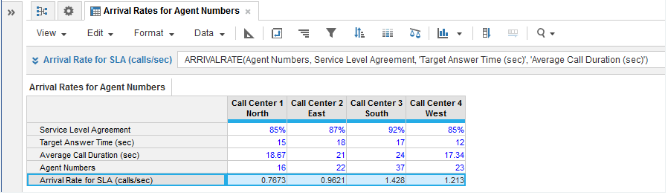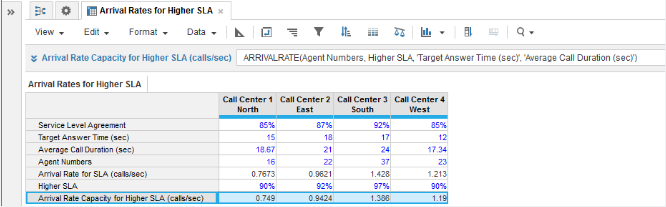1. Calculation functions
2. All Functions
3. Call Center Planning Functions
4. ARRIVALRATECalculates the arrival rate of calls that can be received while guaranteeing the service level agreement.

## Syntax

ARRIVALRATE(x, w, t, z) where:

• x: number of servers, telephone lines, or agents
• w: service level agreement of the percentage of calls to be answered within the target answer time
• z: average call duration

## Format

Input Format Output Format

x: number

w: number (percentage)

t: number (time unit)

z: number (time unit)

Number

## Arguments

The function uses the following arguments:

• x: Numeric line item, property, or expression
• w: Numeric line item, property, or expression
• t: Numeric line item, property, or expression - Must be the same time unit as argument z
• z: Numeric line item, property, or expression - Must be the same time unit as argument t

## Constraints

The function has the following constraints:

• All parameters are mandatory.
• All parameters must be a numeric line item, property, or expression.
• Time unit used for t and z parameters must be the same.
• Result line item must be numeric.
• Maximum number of agents is five million.

## Excel equivalent

• No Excel equivalent

## Example

In this example, we have four call centers each covering a separate geographical area:

• Each call center must achieve a Service Level Agreement (SLA), expressed as the percentage of incoming calls that will be answered for a given target answer time.
• Agents Numbers is a numeric source line item for entering the agent staffing level at each call center.
• Target Answer Time (sec) is a numeric source line item for entering the target answer time for incoming calls.
• Historical monitoring of operational performance provides the average call duration for each call center. This baseline metric is entered in an Average Call Duration (sec) numeric source line item.

We can now use ARRIVALRATE in the Arrival Rate for SLA (calls/sec) numeric result line item to calculate the arrival rate of incoming calls that can be maintained without compromising the agreed SLA.Example 2

In this second example, we use ARRIVALRATE to perform what-if analysis for call arrival rates. Suppose you want to determine the arrival rate of calls that can be handled at your call centers while maintaining a higher SLA. The Higher SLA numeric source line item allows you to enter what-if SLA percentages.

For this example, the SLA has been increased by 5% for each call center. The Arrival Rate Capacity for Higher SLA (calls/sec) numeric result line item provides call arrival rates for increased SLAs.